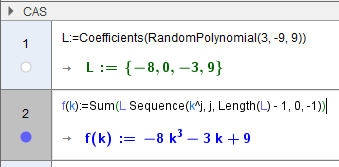# Polynomials displayed in variables other than x

Alex shared this question 2 years ago

Suppose I have a polynomial defined by RandomPolynomial(3, -9, 9), say f(x)=-x^3+x+9.

Is there an easy way to display the same polynomial but using another variable, say, f(k)=-k^3+k+9 ?

Thanks,

Alex1

why should the variable name be relevant?

In CAS you can read the L:=Coefficients( <Polynomial> ) in a list and generate a variable list of P= sequence(k^i, i,0,lenght(L)) and rebuild the polynom as p(k)1

I plan to design some randomized exercises on polynomials, say, asking students to add two random polynomials. So I want some variations, using different variables other than x.

Your method is not perfect for me, because if some coefficients of the polynomial f(x) is 0,1 or -1, sequence(k^i, i,0,lenght(L)) CANNOT give the exact same look as f(x).1

Hm,

L:=Coefficients(RandomPolynomial(3, -9, 9))

h(k):=Sum(L Sequence(k^j, j, Length(L) - 1, 0, -1))what about to change variable by call

h(x):=RandomPolynomial(3, -9, 9)

f(k):=h(k)

this doubles function1

Thanks for reminding me the great CAS. It accepts g(k):=f(k) with given function f(x).1

quote: ...to display the same polynomial but using another variable...

only as text it is possible with custom tool as in the attachment1

Unbelievable! Thanks.1

Thanks ALL. I get want I want: https://www.geogebra.org/m/ftvva88k